Study Materials

NCERT Solutions for Class 11th Mathematics

Page 1 of 5

Chapter 3. Trigonometric Functions

Exercise 3.1

Exercise 3.1

Q1. Find the radian measures corresponding to the following degree measures:

(i) 25°     (ii) - 47°30' (iii) 240°    (iv)  520°

Solution:

(i) 25°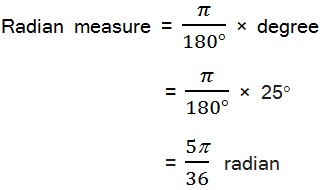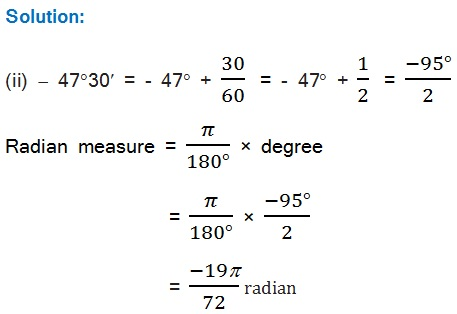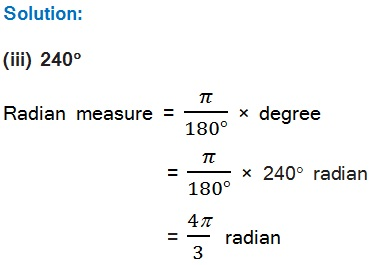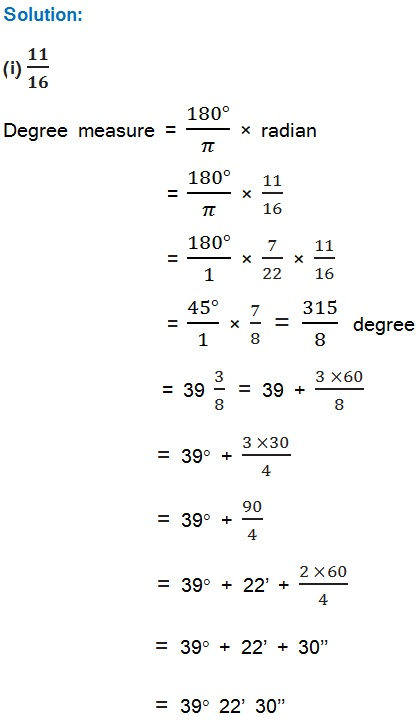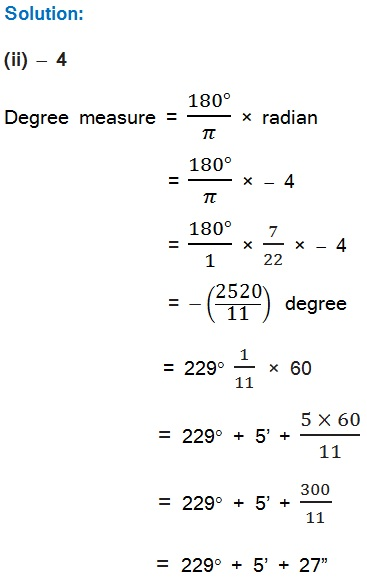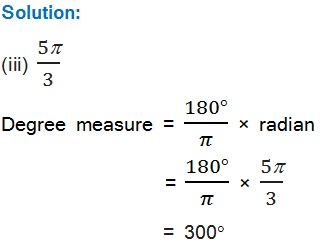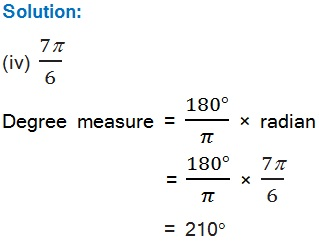Q3. A wheel makes 360 revolutions in one minute. Through how many radians does it turn in one second?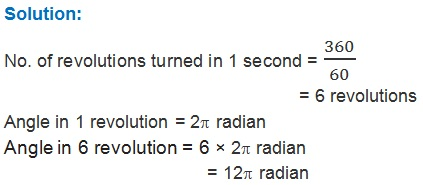Q4. Find the degree measure of the angle subtended at the centre of a circle of radius 100 cm by an arc of length 22 cm (Use π = 22/7).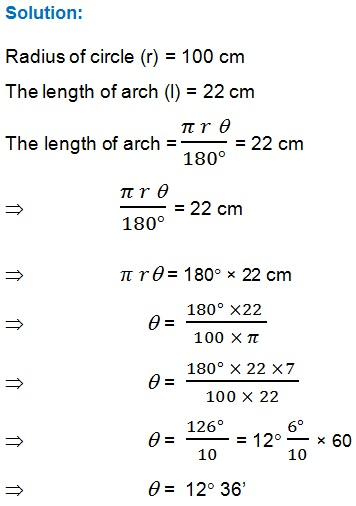Q5. In a circle of diameter 40 cm, the length of a chord is 20 cm. Find the length of minor arc of the chord.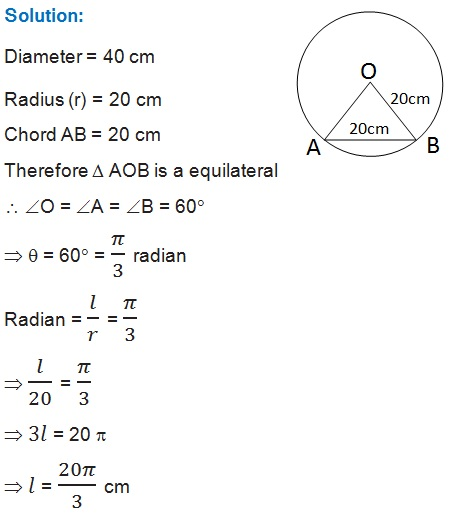Q6. If in two circles, arcs of the same length subtend angles 60° and 75° at the centre, find the ratio of their radii.Q7. Find the angle in radian through which a pendulum swings if its length is 75 cm and the tip describes an arc of length

(i) 10 cm (ii) 15 cm (iii) 21 cm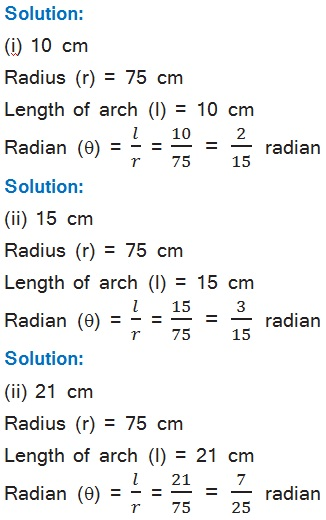Page 1 of 5

Chapter Contents: